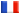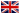# Séminaire de Cryptographie

## Heinrich Matzat### Galois Groups of Additive Polynomials

Additive polynomials over a field $F$ of characteristic $p>0$ have the form $f(X)=\sum\limits^m_{k=0} a_k X^{p^k}$ with $a_k \in F$. In case $a_0 \neq 0$ they are Galois polynomials with an $\mathbb{F}_p$-vector space of solutions, and any finite Galois extension $E$ over $F$ can be generated by such an additive polynomial.

The Galois group of $f(X)$ or $E/F$ , respectively, acts linearly on the solution space and thus is a subgroup of the linear group $\operatorname{GL}_m(\mathbb{F}_p)$. It can be computed via subgroup descent from $\operatorname{GL}_m(\mathbb{F}_p)$ in analogy to the Stauduhar method.

On the other hand, any additive polynomial can be obtained as a characteristic polynomial of a Frobenius module over $F$, i.e., an $F$-vector space $M$ with a $\phi$-semilinear Frobenius operator $\Phi$, where $\phi$ denotes the Frobenius endomorphism of $F$. The smallest connected linear algebraic group in which the representing matrix of $\Phi$ is contained gives an upper bound for the Galois group.

Since lower bounds can be obtained by specialization of the matrix in analogy to the classical Dedekind criterion, this technique gives a useful tool for the construction of Galois extensions with given (connected) Galois group (in positive characteristic). This will be demonstrated by examples, among others the Dickson groups $G_2(q)$.

References:

Goss, D.: Basic structures of function field arithmetic. Springer-Verlag 1996, Chapter I.

Malle, G.: Explicit realization of the Dickson groups $G_2(q)$ as Galois groups. Preprint, Kassel 2002.

Matzat, B. H.: Frobenius modules and Galois groups. Preprint, Heidelberg 2002.